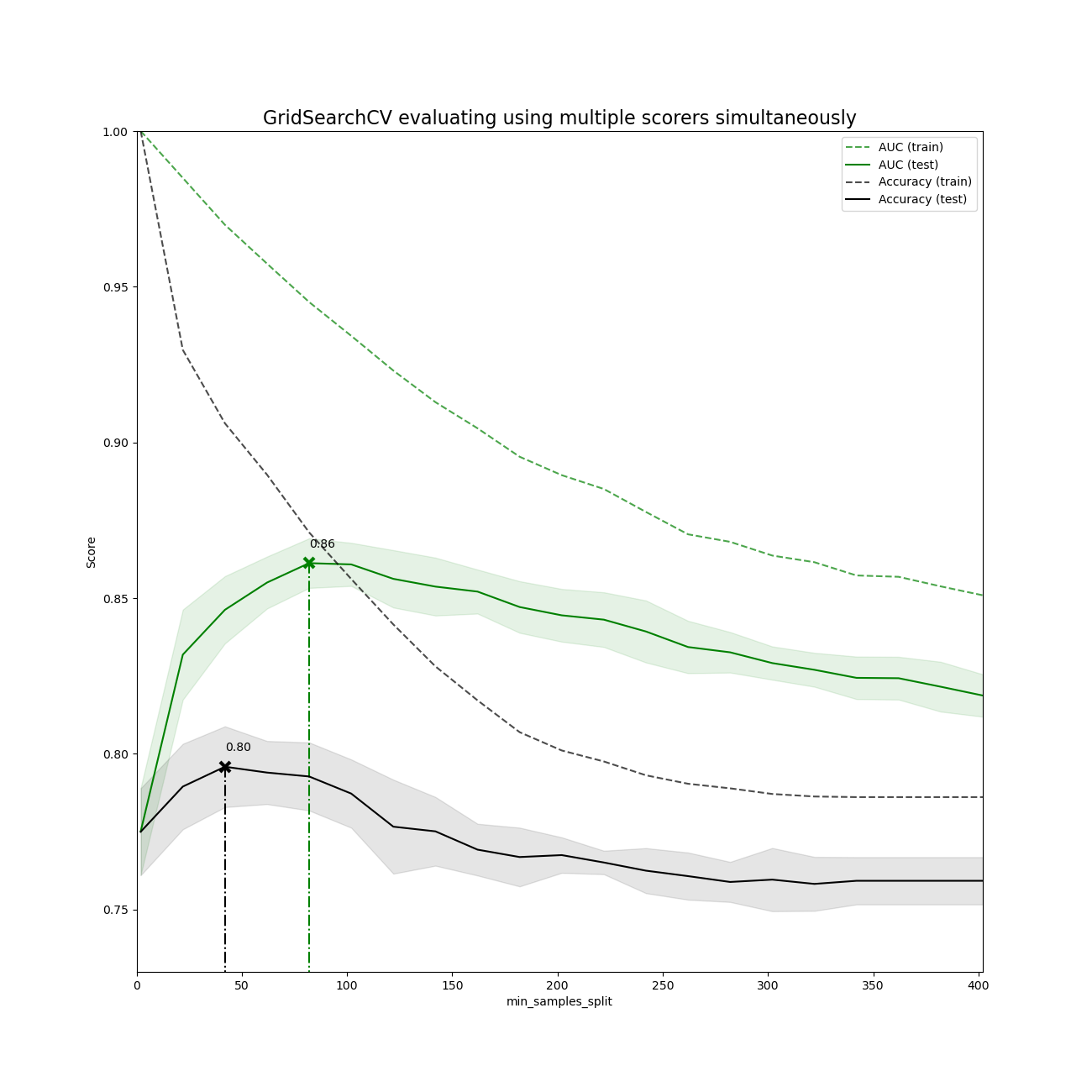# Demonstration of multi-metric evaluation on cross_val_score and GridSearchCV¶

Multiple metric parameter search can be done by setting the scoring parameter to a list of metric scorer names or a dict mapping the scorer names to the scorer callables.

The scores of all the scorers are available in the cv_results_ dict at keys ending in '_<scorer_name>' ('mean_test_precision', 'rank_test_precision', etc…)

The best_estimator_, best_index_, best_score_ and best_params_ correspond to the scorer (key) that is set to the refit attribute.

# Author: Raghav RV <rvraghav93@gmail.com>

import numpy as np
from matplotlib import pyplot as plt

from sklearn.datasets import make_hastie_10_2
from sklearn.metrics import accuracy_score, make_scorer
from sklearn.model_selection import GridSearchCV
from sklearn.tree import DecisionTreeClassifier


## Running GridSearchCV using multiple evaluation metrics¶

X, y = make_hastie_10_2(n_samples=8000, random_state=42)

# The scorers can be either one of the predefined metric strings or a scorer
# callable, like the one returned by make_scorer
scoring = {"AUC": "roc_auc", "Accuracy": make_scorer(accuracy_score)}

# Setting refit='AUC', refits an estimator on the whole dataset with the
# parameter setting that has the best cross-validated AUC score.
# That estimator is made available at gs.best_estimator_ along with
# parameters like gs.best_score_, gs.best_params_ and
# gs.best_index_
gs = GridSearchCV(
DecisionTreeClassifier(random_state=42),
param_grid={"min_samples_split": range(2, 403, 20)},
scoring=scoring,
refit="AUC",
n_jobs=2,
return_train_score=True,
)
gs.fit(X, y)
results = gs.cv_results_


## Plotting the result¶

plt.figure(figsize=(13, 13))
plt.title("GridSearchCV evaluating using multiple scorers simultaneously", fontsize=16)

plt.xlabel("min_samples_split")
plt.ylabel("Score")

ax = plt.gca()
ax.set_xlim(0, 402)
ax.set_ylim(0.73, 1)

# Get the regular numpy array from the MaskedArray
X_axis = np.array(results["param_min_samples_split"].data, dtype=float)

for scorer, color in zip(sorted(scoring), ["g", "k"]):
for sample, style in (("train", "--"), ("test", "-")):
sample_score_mean = results["mean_%s_%s" % (sample, scorer)]
sample_score_std = results["std_%s_%s" % (sample, scorer)]
ax.fill_between(
X_axis,
sample_score_mean - sample_score_std,
sample_score_mean + sample_score_std,
alpha=0.1 if sample == "test" else 0,
color=color,
)
ax.plot(
X_axis,
sample_score_mean,
style,
color=color,
alpha=1 if sample == "test" else 0.7,
label="%s (%s)" % (scorer, sample),
)

best_index = np.nonzero(results["rank_test_%s" % scorer] == 1)
best_score = results["mean_test_%s" % scorer][best_index]

# Plot a dotted vertical line at the best score for that scorer marked by x
ax.plot(
[
X_axis[best_index],
]
* 2,
[0, best_score],
linestyle="-.",
color=color,
marker="x",
markeredgewidth=3,
ms=8,
)

# Annotate the best score for that scorer
ax.annotate("%0.2f" % best_score, (X_axis[best_index], best_score + 0.005))

plt.legend(loc="best")
plt.grid(False)
plt.show()Total running time of the script: (0 minutes 8.376 seconds)

Gallery generated by Sphinx-Gallery